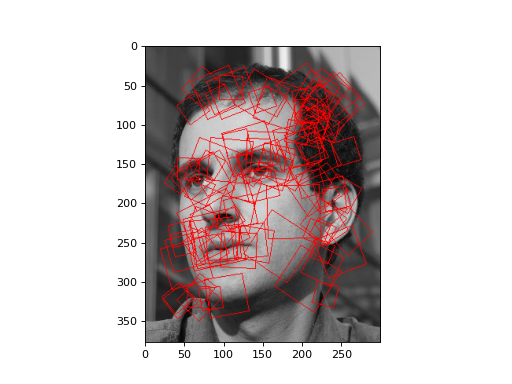# Speeded-Up Robust Features¶

New in version 0.8: In version 0.8, some of the inner functions are now in mahotas.features.surf instead of mahotas.surf

Speeded-Up Robust Features (SURF) are a recent innovation in the local features family. There are two steps to this algorithm:

1. Detection of interest points.
2. Description of interest points.

The function `mahotas.features.surf.surf` combines the two steps:

```import numpy as np
from mahotas.features import surf

f = ... # input image
spoints = surf.surf(f)
print("Nr points: {}".format(len(spoints)))
```

Given the results, we can perform a simple clustering using, for example, milk (nowadays, scikit-learn would be a better choice):

```try:
import milk

# spoints includes both the detection information (such as the position
# and the scale) as well as the descriptor (i.e., what the area around
# the point looks like). We only want to use the descriptor for
# clustering. The descriptor starts at position 5:
descrs = spoints[:,5:]

# We use 5 colours just because if it was much larger, then the colours
# would look too similar in the output.
k = 5
values, _  = milk.kmeans(descrs, k)
colors = np.array([(255-52*i,25+52*i,37**i % 101) for i in xrange(k)])
except:
values = np.zeros(100)
colors = [(255,0,0)]
```

So we are assigning different colours to each of the possible

The helper `surf.show_surf` draws coloured polygons around the interest points:

```f2 = surf.show_surf(f, spoints[:100], values, colors)
imshow(f2)
show()
```

Running the above on a photo of luispedro, the author of mahotas yields:

```from __future__ import print_function
import numpy as np
import mahotas as mh
from mahotas.features import surf
from pylab import *

from os import path

f = f.astype(np.uint8)
spoints = surf.surf(f, 4, 6, 2)
print("Nr points:", len(spoints))

try:
import milk
descrs = spoints[:,5:]
k = 5
values, _  =milk.kmeans(descrs, k)
colors = np.array([(255-52*i,25+52*i,37**i % 101) for i in range(k)])
except:
values = np.zeros(100)
colors = np.array([(255,0,0)])

f2 = surf.show_surf(f, spoints[:100], values, colors)
imshow(f2)
show()
```## API Documentation¶

The `mahotas.features.surf` module contains separate functions for all the steps in the SURF pipeline.

`mahotas.features.surf.``dense`(f, spacing, scale={np.sqrt(spacing)}, is_integral=False, include_interest_point=False)
Parameters: f : image original image spacing : integer Distance between points scale : float, optional Scale of interest points. By default, it is set to `np.sqrt(spacing)` is_integral : boolean, optional Whether f is an integral image include_interest_point : bool, optional Whether to return interest point information. Default is False descriptors : ndarray Descriptors at dense points. Note that the interest point is not returned by default.

`surf`
function Find interest points and then compute descriptors
`descriptors`
function Compute descriptors at user provided interest points
`mahotas.features.surf.``integral`(f, in_place=False, dtype=<type 'numpy.float64'>)

fi = integral(f, in_place=False, dtype=np.double):

Compute integral image

Parameters: f : ndarray input image. Only 2-D images are supported. in_place : bool, optional Whether to overwrite f (default: False). dtype : dtype, optional dtype to use (default: double) fi : ndarray of dtype of same shape as f The integral image
`mahotas.features.surf.``surf`(f, nr_octaves=4, nr_scales=6, initial_step_size=1, threshold=0.1, max_points=1024, descriptor_only=False)

points = surf(f, nr_octaves=4, nr_scales=6, initial_step_size=1, threshold=0.1, max_points=1024, descriptor_only=False):

Run SURF detection and descriptor computations

Speeded-Up Robust Features (SURF) are fast local features computed at automatically determined keypoints.

Parameters: f : ndarray input image nr_octaves : integer, optional Nr of octaves (default: 4) nr_scales : integer, optional Nr of scales (default: 6) initial_step_size : integer, optional Initial step size in pixels (default: 1) threshold : float, optional Threshold of the strength of the interest point (default: 0.1) max_points : integer, optional Maximum number of points to return. By default, return at most 1024 points. Note that the number may be smaller even in the case where there are that many points. This is a side-effect of the way the threshold is implemented: only `max_points` are considered, but some of those may be filtered out. descriptor_only : boolean, optional If `descriptor_only`, then returns only the 64-element descriptors (default is `False`). points : ndarray of double, shape = (N, 6 + 64) N is nr of points. Each point is represented as (y,x,scale,score,laplacian,angle, D_0,...,D_63) where y,x,scale is the position, angle the orientation, score and laplacian the score and sign of the detector; and D_i is the descriptor If `descriptor_only`, then only the D_is are returned and the array has shape (N, 64)!

References

Herbert Bay, Andreas Ess, Tinne Tuytelaars, Luc Van Gool “SURF: Speeded Up Robust Features”, Computer Vision and Image Understanding (CVIU), Vol. 110, No. 3, pp. 346–359, 2008